﻿ Calculation method > SUI

# STANFORD UNIVERSITY INTERIM

Navegación:  Calculation method > SUI

# STANFORD UNIVERSITY INTERIM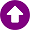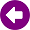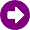DESCRIPTION

The 802.16 IEEE group, jointly with the Stanford University, carried out an extensive work with the aim to develop a channel model for WiMAX applications in suburban environments. One of the most important results obtained was the SUI (Stanford University Interim) propagation loss model , which is an extension of an early work carried out by AT&T Wireless and the further analysis done by Erceg et al. .

To calculate the median path loss using the SUI model, the environment is categorised in three different groups with their own characteristics:

Category A: hilly terrain with moderate-to-heavy tree densities, which results in the maximum path loss.

Category B: hilly environment but rare vegetation, or high vegetation but flat terrain. Intermediate path loss condition is typical of this category.

Categoría C: zonas llanas con densidad de vegetación muy baja o nula. Corresponde a trayectos donde las pérdidas son bajas.

Typically, for the three previous categories, the general scenario is as follows:

Cells size < 10 km.

Receiver antenna height: 2 to 10 m.

Base station antenna height: 15 a 40 m.

High cell coverage requirement (80%-90%).

According to IEEE 802.16 documentation, the SUI model is a suitable propagation model for WIMAX and BFWA (Broadband Fixed Wireless Applications) implementations.

In the following section, the SUI model and some variations including correction factors are described.

DEVELOPMENT

Basic SUI model (or Erceg model)

The basic SUI model was proposed by the IEEE and is based on Erceg’s model , for frequencies around 2 GHz, receiver antenna below 2 m. It is suitable to suburban environments.

The median path loss is defined as the following expression: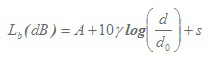(1)

where:

 d > d0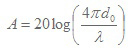(2)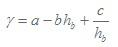(3)
 d0 = 100 m 10 m < hb < 80 m 8.2 dB < s < 10.6 dB

d: distance between base station and receiver, in m.

λ: wavelength, in m; f ≤ 2000 MHz.

γ: path-loss exponent.

hb: base station height, in m.

a, b, c: constants dependent on the terrain category (see Table 1).

 Categoría A Categoría B Categoría C a 4,6 4 3,6 b 0,0075 0,0065 0,005 c 12,6 17,1 20

Tabla 1: SUI model parameters.

SUI Model with correction factors

For frequencies above 2 GHz and receiver antenna heights, h, between 2 and 10 m, several correction factors are introduced in the above expression (1), namely: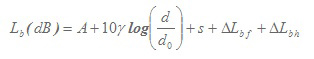(4)

where:

ΔLbf: frequency correction factor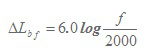(5)

ΔLbh: receiver antenna height correction factor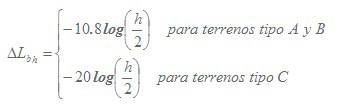(6)

This version of the SUI model, including correction factors, is the most employed in path loss estimation using this model.

Extended SUI Model

The extended SUI model  proposed by the IEEE 802.16 modifies the correction factor ΔLbh in (6), according to that proposed by Okumura. This modification introduces a new calculation procedure of the reference distance d0. The new distance is, therefore, d’0, and its value is given by (9). Thus, the following expressions are defined as the method to estimate the median path loss: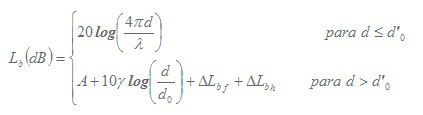(7)

where: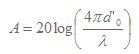(8)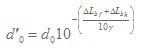(9)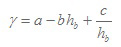(10)

ΔLbf: frequency correction factor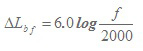(11)

ΔLbh: receiver antenna height correction factor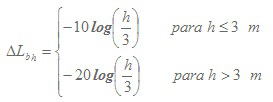(12)

The parameters a, b, c and d0 of the model, are calculated using the procedure defined in the previous section (see Table 1).

REFERENCES

 V. Erceg et al., "An empirically-based path loss model for wireless channels in suburban environments," Global Telecommunications Conference, 1998. GLOBECOM 1998. The Bridge to Global Integration. IEEE, Sydney, NSW, 1998, pp. 922-927 vol.2. doi: 10.1109/GLOCOM.1998.776865

 V. Erceg et al., "Channel Models for Fixed Wireless Applications", IEEE802.16.3c-01/29r4, Broadband Wireless Working Group, IEEE P802.16, 2001.

 G. Senarath, W. Tong et. al: "Multi-hop Relay System Evaluation Methodology (Channel Model and Performance Metric)", IEEE 802.16j-06/013r3, Feb 2007.